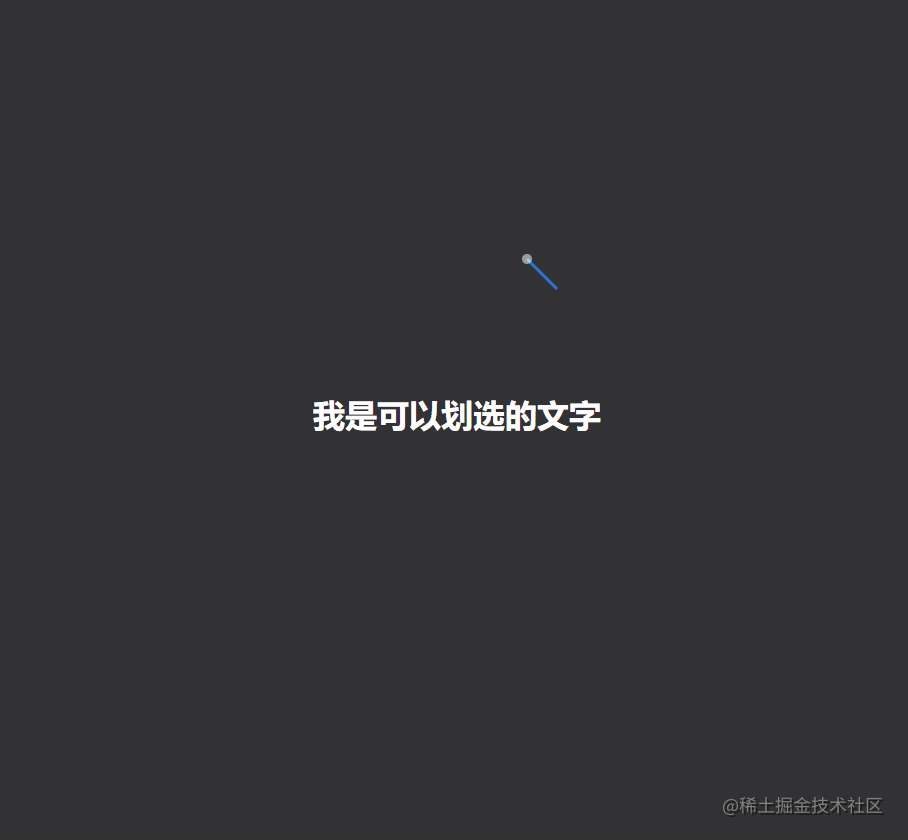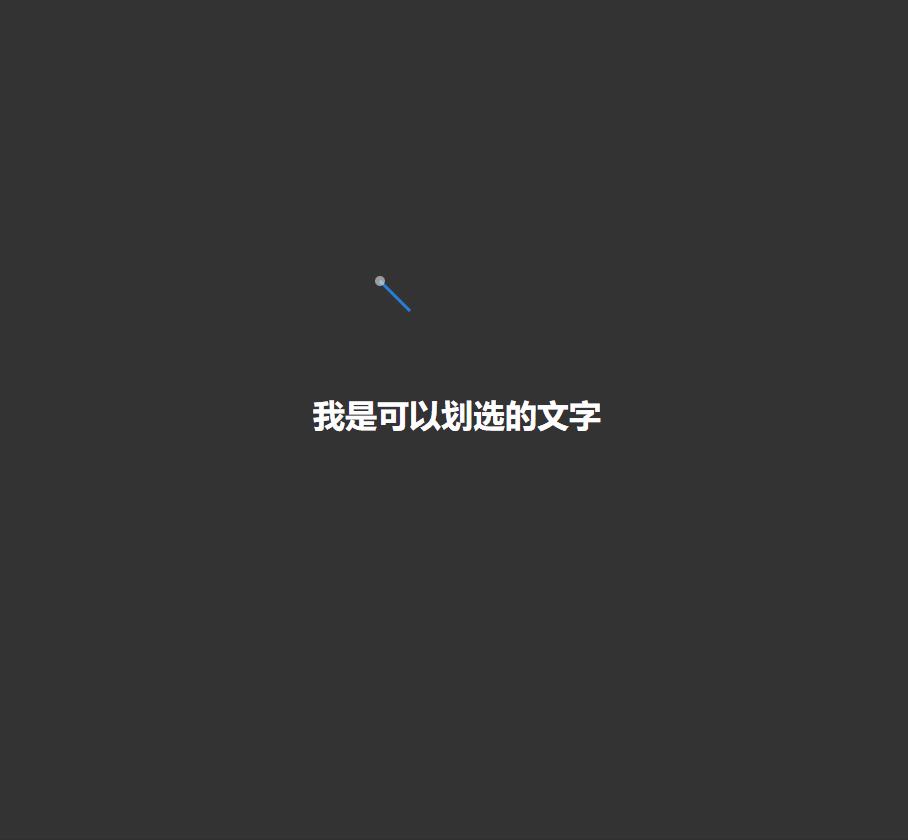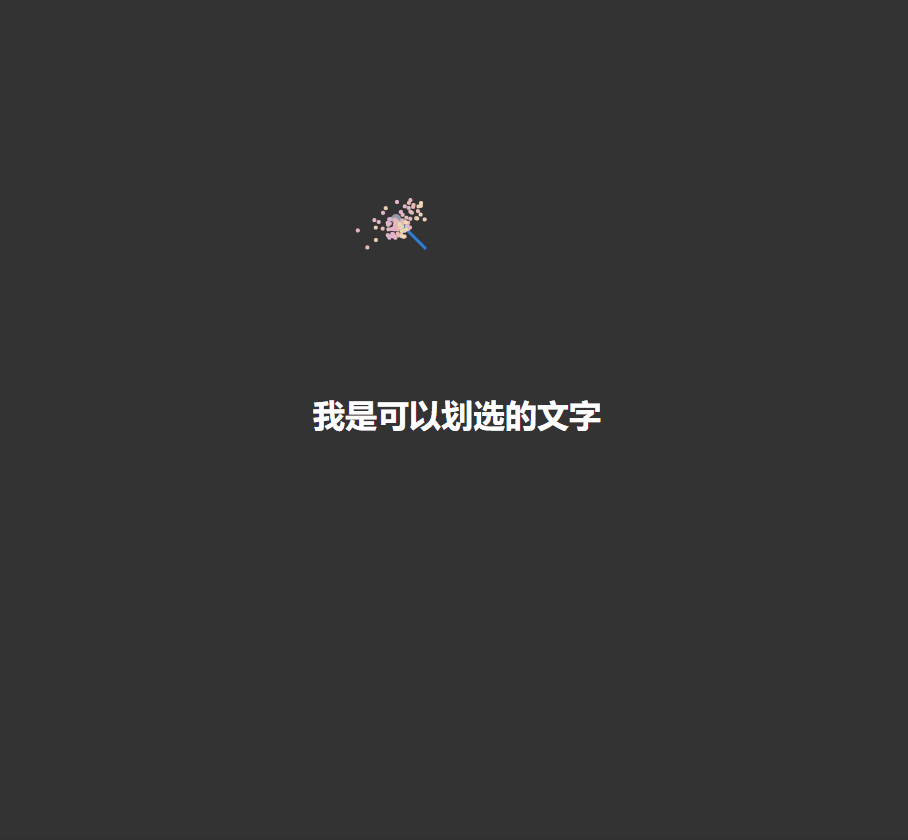# 我是如何用 canvas 实现一个自定义仙女棒的？### 前言### 自定义鼠标样式

``````*{margin: 0; padding: 0;}
body {
display: flex;
width: 100%;
height: 100vh;
overflow: hidden;
background: #333;
align-items: center;
justify-content: center;
}

`css` 代码很简单，只有几行，当然这只是这个 `demo` 中添加的样式，真实的开发中就可以安装你们自己的需求来编写相关的 `css` 代码了。

``````class CustomMouse {
canvas: HTMLCanvasElement;
ctx: CanvasRenderingContext2D;
mouse: { x: number; y: number; };
backgroundColor: string;
particles: Particle[];
constructor() {
this.canvas = document.createElement('canvas');
document.body.appendChild(this.canvas);
this.canvas.style.position = 'fixed';
this.canvas.width = innerWidth;
this.canvas.height = innerHeight;
this.canvas.style.zIndex = '999';
this.canvas.style.pointerEvents = 'none';
this.ctx = this.canvas.getContext('2d');
this.backgroundColor = 'rgba(30, 144, 255, 0.8)';
this.mouse = { x: -100, y: -100 };
this.particles = [];

this.init();
this.animate();
}
init() {
this.ctx.fillStyle = this.backgroundColor;
this.ctx.strokeStyle = this.backgroundColor;

document.styleSheets.insertRule(`* {cursor: none;}`, 0);
}
}

``````class CustomMouse {
...other code

init() {
...other code

this.event();
}

event() {
this.mouse.x = evt.x;
this.mouse.y = evt.y;
});
}

animate() {
requestAnimationFrame(() => this.animate());
this.ctx.clearRect(0, 0, this.canvas.width, this.canvas.height);
this.stick();
}

stick() {
const { x, y } = this.mouse;
this.ctx.beginPath();
this.ctx.moveTo(x, y);
this.ctx.lineTo(x + 30, y + 30);
this.ctx.lineWidth = 3;
this.ctx.stroke();
this.ctx.fillStyle = `rgba(255, 255, 255, .5)`;
this.ctx.beginPath();
this.ctx.arc(x, y, 5, 0, Math.PI * 2);
this.ctx.fill();
this.ctx.fillStyle = this.backgroundColor;
}
}### 跟随鼠标的粒子

``````class Particle {
x: number;
y: number;
vx: number;
vy: number;
age: number;
ctx: CanvasRenderingContext2D;
color: string;
constructor(x: number, y: number, ctx: CanvasRenderingContext2D, mouseClick?: boolean) {
this.x = x;
this.y = y;
if (!mouseClick) {
this.vx = Math.random() - 0.5;
this.vy = Math.random();
this.age = Math.random() * 30;
} else {
let deg = Math.random() * Math.PI * 2;
let r = Math.random();
this.vx = r * Math.cos(deg);
this.vy = r * Math.sin(deg);
this.age = Math.random() * 30 + 60;
}
this.ctx = ctx;
this.color = `hsl(\${this.randomColor() * this.vx}, 50%, 80%)`;
}
update() {
this.age--;
this.x += this.vx;
this.y += this.vy;
}
draw() {
this.ctx.beginPath();
this.ctx.arc(this.x, this.y, 2, 0, Math.PI * 2);
this.ctx.fillStyle = this.color;
this.ctx.fill();
}
randomColor() {
return Math.random() * 255 | 0;
}
}

`Particle` 类中，唯一需要注意的点就是在 `constructor` 方法中判断当前是鼠标移动还是鼠标点击，这是因为我们要复用这个粒子类来做鼠标和点击不同的效果，当然这里面也用到了三角函数相关的知识点，如果有对三角函数还不了解的同学，可以点击这里查看之前的文章。

``````class CustomMouse {
...other code

event() {
this.mouse.x = evt.x;
this.mouse.y = evt.y;
for (let i = 0; i < 30; i++) {
this.particles.push(new Particle(this.mouse.x, this.mouse.y, this.ctx));
}
});
}

animate() {
...other code

for (let i in this.particles) {
const p = this.particles[i];
p.update();
p.draw();
if (p.age < 0) {
this.particles.splice(i as unknown as number, 1);
}
}
}
}``````class CustomMouse {
...other code

event() {
...other code

for (let i = 0; i < 100; i++) {
this.particles.push(new Particle(this.mouse.x, this.mouse.y, this.ctx, true));
}
});
}
}

### 往期回顾

canvas 实现多彩的圆环数字时钟

canvas 实现燃烧的线段，原来线段也能玩的这么出彩

canvas 动画真好玩，快来学一下这炫酷的效果吧！